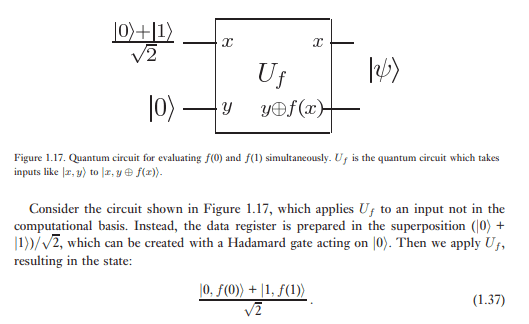# [Quantum Computing] Quantum Parallelism State Calculation

• I
llha
TL;DR Summary
Nielsen and Chuang state calculation isn't the full tensor product? But a full tensor product would be useless to measure?
Hi, I'm going through Nielsen and Chuang's Quantum Computation and Quantum Information textbook and I don't really understand this part about quantum parallelism:Shouldn't the resulting state be (1/sqrt(2^4)) * (|0, f(0)> + |0, f(1)> + |1, f(1)> + |1, f(0)>), since the resulting state would be the (normalized) tensor product of (1/sqrt(2)) * (|0> + |1>) and (1/sqrt(2)) * (|f(0)> + f(1)>)?

I understand that would be pretty useless to measure, so I know I'm wrong, but I don't understand where I'm going wrong. Thanks in advance.